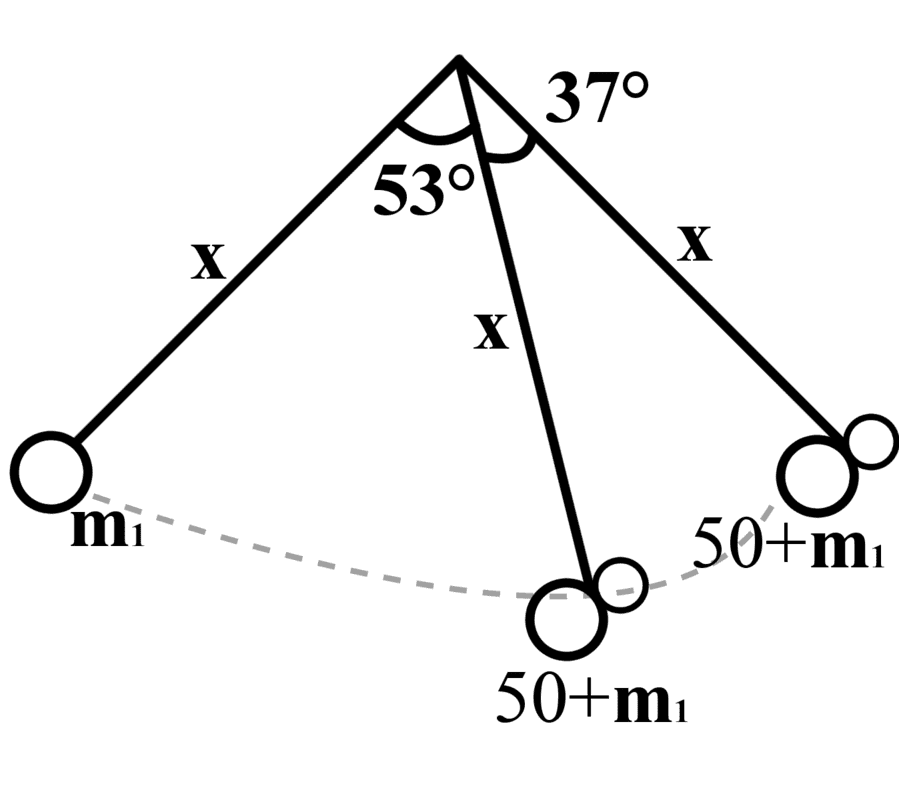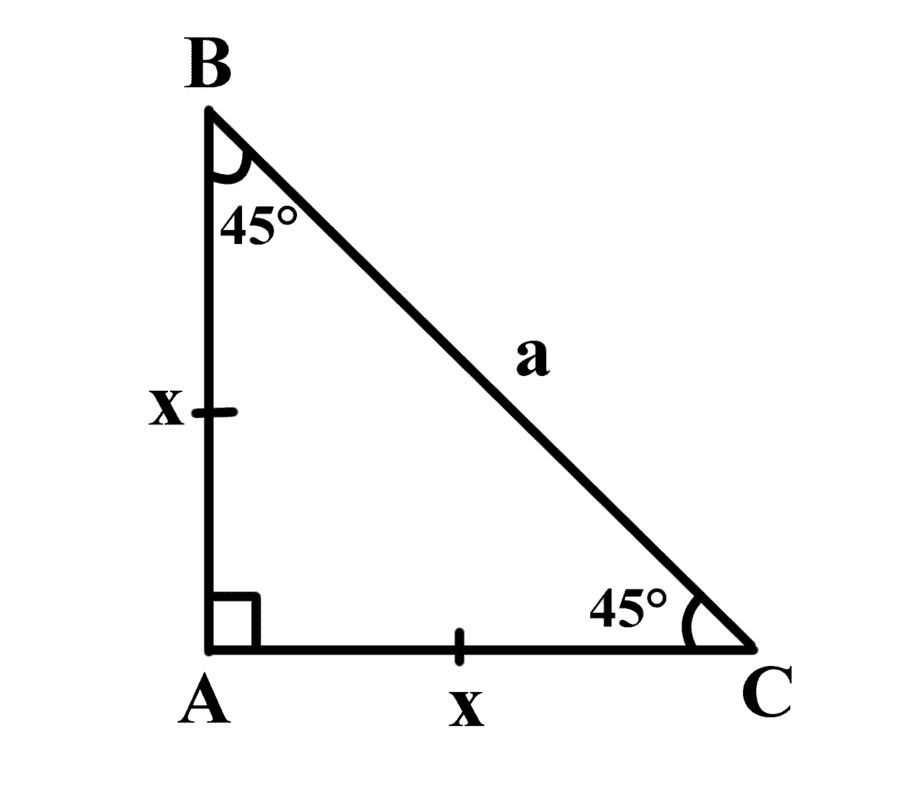# Finding the mass of Tarzan?

Homework Statement:
Jane thinks that Tarzan has been gaining too much mass. However, he doesn’t want to tell her what he weighs. One day Tarzan is swinging in the forest. He starts from rest from one side at an angle of 53° from the vertical. He swings down and grabs Jane at the bottom of the swing. They swing together on the other side up to a maximum angle of 37 °. Jane knows that her mass is 50 kg. Calculate Tarzan’s mass. (Ans.120.8kg)
Relevant Equations:
Refer Below.
My Attempt So Far:

1) Drawing a diagram based on the question.Diagram 1: Pendulum Diagram Based On Question

2) List down pieces of information found in the question.

θ1=53°
θ2=37°
m1=m1
m2=m1+50kg
v
i=0

3) Since 53°+37°=90°, I redraw another diagram making it into a right angle triangle in order to find the length of the vine.
Since the length of the vine is the same in the initial location and final location, I assumed that the adjacent and opposite sides are equal.Diagram 2: Right-Angled Triangle Derived From Question

4) I then tried to find the length of x by applying the Cosine Rule.

c2=a2+b2-2ab(cos C)
Equation 1: Cosine Rule

x2=a2+x2-2ax(cos 45)

Substitute the unknowns with the numbers or unknowns that are shown in the diagram drawn.

-a2=-2ax(cos 45)
Cancel like terms and try to put everything in term of a

a=√2 x
Let this equation be the first equation.

5) After getting the first equation, I tried finding the length of the vine by using sin θ = Opposite/Hypotenuse

sin 45 = x/a

sin 45 = x/√2 x

sin 45 = √2/2

√2=x

2=a

I was stuck from this point on... Any help is appreciated...

•Delta2

PeroK
Homework Helper
Gold Member
2020 Award
Why not let the length of the vine be ##l## and see what you get. Perhaps ##l## will cancel out.

Hint: think about conservation of momentum and energy. Where and when do these apply in this problem?

Last edited:
Doc Al
Mentor
In addition to following @PeroK's advice about the length of the vine, compare the situation immediately before and immediately after Tarzan grabs Jane. What's different? What remains the same?

So I am assuming that the initial position has mgh,
Why not let the length of the vine be ##l## and see what you get. Perhaps ##l## will cancel out.

Hint: think about conservation of momentum and energy. Where and when do these apply in this problem?

So, at the initial position, there is mgh, but no 1/2mv2? Since it started at rest? then at the middle point when he grabbed Jane, the mgh is 0? After that I am not sure

In addition to following @PeroK's advice about the length of the vine, compare the situation immediately before and immediately after Tarzan grabs Jane. What's different? What remains the same?

The mass and velocity? I am assuming that as the mass increase the velocity decreases?

Doc Al
Mentor
So, at the initial position, there is mgh, but no 1/2mv2? Since it started at rest? then at the middle point when he grabbed Jane, the mgh is 0?
Yes, at the initial and final positions, there is potential energy but no kinetic. And it's OK to measure PE from the lowest point.

PeroK
Homework Helper
Gold Member
2020 Award
So I am assuming that the initial position has mgh,

So, at the initial position, there is mgh, but no 1/2mv2? Since it started at rest? then at the middle point when he grabbed Jane, the mgh is 0? After that I am not sure
In physics terms what can you say about the process of Tarzan picking up Jane? Is energy conserved? Is momentum conserved?

In physics terms what can you say about the process of Tarzan picking up Jane? Is energy conserved? Is momentum conserved?

So, um Energy cannot be destroyed but it can be transfer? I assume the momentum is conserved as the momentum before and the momentum after is equal when there are no external forces

Yes, at the initial and final positions, there is potential energy but no kinetic. And it's OK to measure PE from the lowest point.

By that, since the energy is conserved, I can assume that the PE of initial and final to be equal right?

Doc Al
Mentor
Consider this in three parts: The swing down from the starting point to the bottom; The "collision" as Tarzan grabs Jane (what kind of collision is this?); The swing up to the final height.

In each of these parts, some quantities are conserved while others are not.

•PeroK
Consider this in three parts: The swing down from the starting point to the bottom; The "collision" as Tarzan grabs Jane (what kind of collision is this?); The swing up to the final height.

In each of these parts, some quantities are conserved while others are not.
When Tarzan grab Jane, it stick together, so it would be... perfect inelastic collision?

•PeroK
Doc Al
Mentor
When Tarzan grab Jane, it stick together, so it would be... perfect inelastic collision?
Exactly! Make use of that insight.

Consider this in three parts: The swing down from the starting point to the bottom; The "collision" as Tarzan grabs Jane (what kind of collision is this?); The swing up to the final height.

In each of these parts, some quantities are conserved while others are not.
Exactly! Make use of that insight.
so... V2=[m1/(m1+m2)]v1

(Sorry, I dont know how to use fraction here...)

so... V2=[m1/(m1+m2)]v1

(Sorry, I dont know how to use fraction here...)

PeroK
Homework Helper
Gold Member
2020 Award
Can you relate ##v_1## and ##v_2## to the initial and final positions?

•Doc Al
Can you relate ##v_1## and ##v_2## to the initial and final positions?
##v_1## the initial velocity is at zero, since it's stated that Tarzan was at rest...
##v_2## the final velocity is it zero too? Since he stopped?

(I am really sorry for taking so much of both of your time...)

Doc Al
Mentor
##v_1## the initial velocity is at zero, since it's stated that Tarzan was at rest...
##v_2## the final velocity is it zero too? Since he stopped?
##v_1## and ##v_2## are the velocities immediately before and after the inelastic collision.

##v_1## and ##v_2## are the velocities immediately before and after the inelastic collision.
Hmm... This is slightly confusing...

##v_1## and ##v_2## are the velocities immediately before and after the inelastic collision.
So there will be a ##v_f## for final velocity of both right?

Doc Al
Mentor
So there will be a ##v_f## for final velocity of both right?
In your momentum equation, that "final" velocity is ##v_2##.

In your momentum equation, that "final" velocity is ##v_2##.
##v_2##=[##m_1##/(##m_1##+##m_2##)]##v_1##
This equation?

How do I use this to obtain the final velocity when I don't know the ##v_1##?

Doc Al
Mentor
##v_2##=[##m_1##/(##m_1##+##m_2##)]##v_1##
This equation?

How do I use this to obtain the final velocity when I don't know the ##v_1##?
As @PeroK suggested, express those velocities in terms of the initial and final positions. (What's conserved during those swings?)

As @PeroK suggested, express those velocities in terms of the initial and final positions. (What's conserved during those swings?)
The momentum is conserved?
Position as in displacement?

PeroK
Homework Helper
Gold Member
2020 Award
##v_1## the initial velocity is at zero, since it's stated that Tarzan was at rest...
##v_2## the final velocity is it zero too? Since he stopped?

(I am really sorry for taking so much of both of your time...)
To give you a bit more help, let me show you how I would set up this problem. There are three phases.

The first phase is Tarzan swinging. Energy is conserved. Let's say the energy is ##E_1##. We start with GPE and end with KE.

The second phase he collides with Jane and energy is lost. After the collision the energy is ##E_2##.

We can relate ##E_1## and ##E_2## by conservation of momentum.

The third phase is both swinging up. Again energy is conserved and KE becomes GPE.

Note: you have to be careful if you like using "initial" and "final" terminology. These apply to each phase of the motion. Especially for more complicated problems I prefer using ##v_0, v_1, v_2, v_3## etc.

In this problem ##v_0 = 0## and ##v_3 = 0##, so we don't even need those.

But we do need ##v_1## and ##v_2##, as the velocities just before and after the collision.

To give you a bit more help, let me show you how I would set up this problem. There are three phases.

The first phase is Tarzan swinging. Energy is conserved. Let's say the energy is ##E_1##. We start with GPE and end with KE.

The second phase he collides with Jane and energy is lost. After the collision the energy is ##E_2##.

We can relate ##E_1## and ##E_2## by conservation of momentum.

The third phase is both swinging up. Again energy is conserved and KE becomes GPE.

Note: you have to be careful if you like using "initial" and "final" terminology. These apply to each phase of the motion. Especially for more complicated problems I prefer using ##v_0, v_1, v_2, v_3## etc.

In this problem ##v_0 = 0## and ##v_3 = 0##, so we don't even need those.

But we do need ##v_1## and ##v_2##, as the velocities just before and after the collision.
So.
##E_1##=##m##g##h##+½mv12
##E_2## = ½(m+50) v22

then
##E_1## = ##E_2##